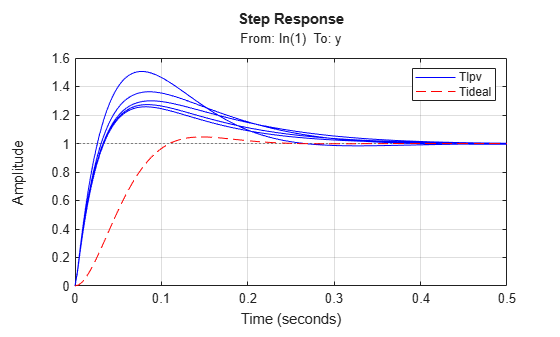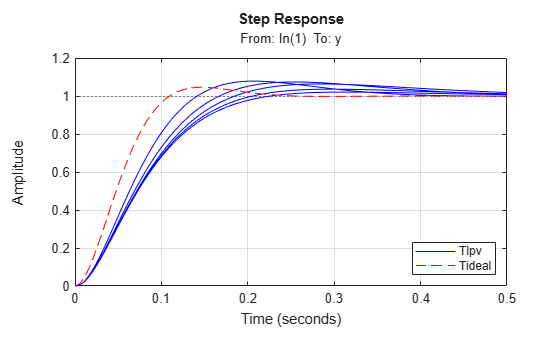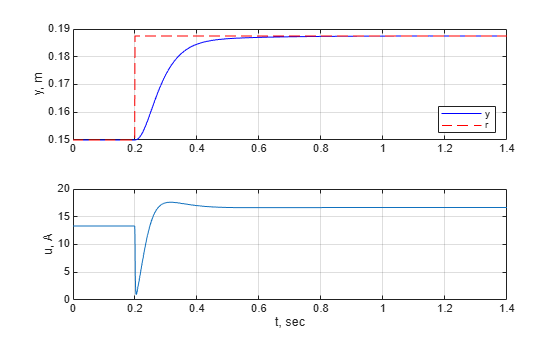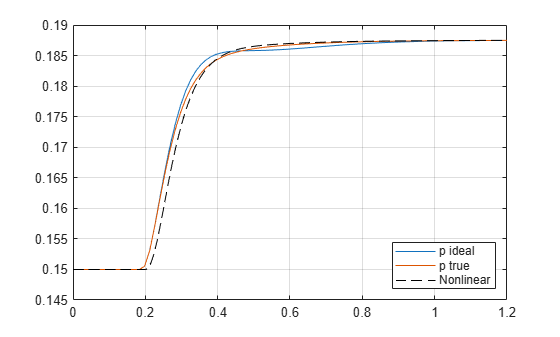# LPV Model of Magnetic Levitation Model from Batch Linearization Results

This example shows how to obtain a linear parameter-varying model of a nonlinear model for a magnetic levitation system from batch linearization results. This nonlinear model is described in the LPV Model of Magnetic Levitation System example.

In this example, you:

1. Linearize a nonlinear Simulink® model on a grid of trim points.

2. Construct an LPV model from the gridded array of LTI models and trim offsets.

3. Design a controller on a grid of trim points. This grid may be different from the grid used for the linearization.

4. Test the controller using command-line LTI simulations (at frozen operating points) and LPV simulations (along a specified parameter trajectory).

5. Test the controller on the nonlinear plant in Simulink.

### Model Parameters

`open_system('MaglevOpenLoop.slx')`Specify the model parameters.

```mb = 0.02; g = 9.81; alpha = 2.4832e-5; umin = -inf; umax = inf;```

### Batch Trim and Linearization

Specify trim conditions as five different height values: ($\mathit{h}$,$\stackrel{˙}{\mathit{h}}$) = (${\mathit{h}}_{\mathit{s}}\left(\mathit{i}\right)$,0).

```Ns = 5; hmin = 0.05; hmax = 0.25; hs = linspace(hmin,hmax,Ns);```

Create operating point specifications.

```clear opspec; for i=1:Ns % Set states to desired equilibrium values h0 = hs(i); hdot0 = 0; % Initialize operating point specification opspec(i) = operspec('MaglevOpenLoop'); % Position: h=hs(i) opspec(i).States(1).Known = 1; opspec(i).States(1).SteadyState = 1; % Velocity: hdot=0 opspec(i).States(2).Known = 1; opspec(i).States(2).SteadyState = 1; % Input current: Restrict u>=0 opspec(i).Inputs.Min=0; end```

Find trim points.

```opt = findopOptions('DisplayReport','off', 'OptimizerType','graddescent-proj'); [op,report] = findop('MaglevOpenLoop',opspec,opt);```

Compute linearizations.

```io = [linio('MaglevOpenLoop/u - Current [A]',1,'in'); % u linio('MaglevOpenLoop/Magnetic Levitation Plant Model',1,'out')]; % y linOpt = linearizeOptions('StoreOffsets',true); [G,~,info] = linearize('MaglevOpenLoop',op,io,linOpt); G.u = 'u'; G.y = 'y'; G.SamplingGrid = struct('h',hs);```

Construct the LPV model using `ssInterpolant`.

```offsets = info.Offsets; Glpv = ssInterpolant(G,offsets);```

### Batch PID Tuning

The grid for the controller is coarser than the one used for the model to illustrate that you can do so. Moreover, the controller gridding is often coarse to simplify the controller design (fewer grid points) while allowing a higher-fidelity LPV model with finer gridding for analysis and simulation.

```Ncd = 3; hcd = linspace(hmin,hmax,Ncd);```

Define `Tideal` as the ideal, second-order response corresponding to (${\omega }_{\mathit{n}}$,$\zeta$).

```wn = 30; zeta = 0.7; Tideal = tf(wn^2,[1 2*zeta*wn wn^2]);```

Use `pidtune` to tune a gain-scheduled PID controller.

```wc = 50; [Ga,Goffsets] = sample(Glpv,[],hcd); Ka = pidtune(Ga,'pid',wc); Ka.Tf = 0.001; Ka.SamplingGrid.h = hcd;```

Build an LPV controller with equations

`$\stackrel{˙}{\zeta }={\mathit{A}}_{\mathit{K}}\left(\mathit{p}\right)\zeta +{\mathit{B}}_{\mathit{K}}\left(\mathit{p}\right)\mathit{e}$`

`$\mathit{u}={\mathit{u}}_{0}\left(\mathit{p}\right)+{\mathit{C}}_{\mathit{K}}\left(\mathit{p}\right)\zeta +{\mathit{D}}_{\mathit{K}}\left(\mathit{p}\right)\mathit{e},$`

where ${\mathit{u}}_{0}\left(\mathit{p}\right)$is the trim current for the height $\mathit{p}$. This controller regulates the current around this equilibrium value.

```Koffsets = struct('u',0,'y',{Goffsets.u}); Ka = ss(Ka); Klpv = ssInterpolant(Ka,Koffsets);```

### LTI Analysis

Simulate the step response of the closed-loop model and compare with `Tideal`.

```figure(1); Tf = 0.5; Tlpv = feedback(Glpv*Klpv,1); step(sample(Tlpv,[],hs),'b',Tideal,'r--',Tf); grid on; legend('Tlpv','Tideal');```The closed-loop system has additional zeros not in the ideal response `Tideal`. These zeros arise from the PID controller and cause a shorter rise time and larger overshoot.

You can use a two degree-of-freedom architecture with a reference prefilter `Fref` to reduce the overshoot. Model the prefilter as an LTI system for simplicity.

```Fref = ss(-10,10,1,0); figure(2); Tf = 0.5; step(sample(Tlpv*Fref,[],hs),'b',Tideal,'r--',Tf); grid on; legend('Tlpv','Tideal','Location','Southeast');```### Compare LPV and Nonlinear Simulations

Simulate the nonlinear response with the gain-scheduled PID. The `MaglevClosedLoop.slx` model uses the LPV System block for the controller.

Specify initial conditions and simulate the model.

```h0 = (hmin+hmax)/2; hdot0 = 0; tstep = 0.2; Tf = 1.2; hstep0 = h0; hStepAmp = 0.25*h0; hstepf = h0+hStepAmp; sim('MaglevClosedLoop',[0 tstep+Tf]);```

Plot the responses.

```figure(3); subplot(2,1,1); plot(y.Time,y.Data,'b',r.Time,r.Data,'r--'); ylabel('y, m'); legend('y','r','Location','Southeast'); grid on; subplot(2,1,2); plot(u.Time,u.Data); ylabel('u, A'); xlabel('t, sec'); grid on;```Compare results with the LPV simulation.

`CL = Tlpv*Fref;`

Set $p\left(t\right)$ to an ideal trajectory and simulate the step response.

```t = linspace(0,tstep+Tf,100); StepConfig = RespConfig('InputOffset',h0,'Delay',tstep,'Amplitude',hStepAmp); p_ideal = step(Tideal*Fref,t,StepConfig); y1 = step(CL,t,p_ideal,StepConfig);```

Set $p\left(t\right)=h\left(t\right)$ and simulate the step response.

```pFcn = @(t,x,u) x(1); StepConfig = RespConfig('InputOffset',h0,'Delay',tstep,... 'Amplitude',hStepAmp,'InitialParameter',h0); y2 = step(CL,t,pFcn,StepConfig);```

Plot the response comparison.

```figure(4) tsim = y.Time; ysim = y.Data; plot(t,y1,t,y2,tsim,ysim,'k--'); legend('p ideal','p true','Nonlinear','Location','Southeast'); xlim([0 Tf]), grid```Both LPV simulations approximate the nonlinear response well. The simulation with the true trajectory provides a slightly better approximation.

Close the models.

```bdclose('MaglevOpenLoop') bdclose('MaglevClosedLoop')```